Courses

# Logical Effort of Multistage Logic Networks Electrical Engineering (EE) Notes | EduRev

## Electrical Engineering (EE) : Logical Effort of Multistage Logic Networks Electrical Engineering (EE) Notes | EduRev

The document Logical Effort of Multistage Logic Networks Electrical Engineering (EE) Notes | EduRev is a part of Electrical Engineering (EE) category.
All you need of Electrical Engineering (EE) at this link: Electrical Engineering (EE)

Objectives

In this lecture you will learn the following

• Logical Effort of Multistage Logic Networks
• Minimizing Delay along a Path
• Few Examples

23.1 Logical Effort of Multistage Logic Networks

The logical effort along a path compounds by multiplying the logical effort of all the logic gates along the path.We denote it by the letter 'G'. Hence,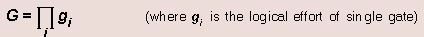The electrical effort along a path through the network is simply the ratio of the capacitance that loads the logic gate in the path to input capacitance of the first gate in the path.We denote it by the letter 'H' .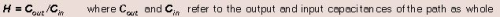When fanout occurs within a logic network, some of the available drive current is directed along the path we are analyzing, and some are directed off that path. Branching effort (b) at the output of a logic gate is defined as-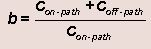where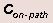is the load capacitance along the path and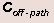is the capacitance of connections that lead off the path.If there is no branching in the path the branching effort is unity.

Branching effort along the entire path 'B' is the product of branching effort at each of the stages along the path.-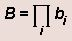Path effort(F) is defined as -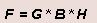The path branching and electrical effort are related to the electrical effort of each stage as-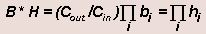The path delay D is the sum of the delays of each of the stages of logic in the path.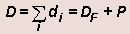where DF is path effort delay and P is path parasitic delay which are given as -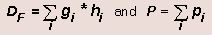23.2 Minimizing Delay along a Path

Consider two path stages as in figure 23.21.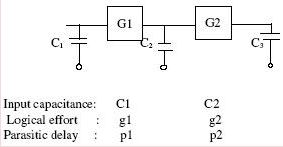Fig 23.21: An Example Circuit

The total delay of the above circuit is given by-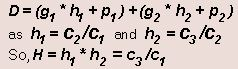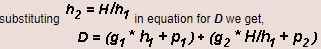To minimise D , we take the partial derivative of D with respect to equating it to zero we get,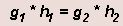i.e. the product of logical effort and electrical effort of each stage should be equal to get minimum delay.This is independent of scale of circuit and of the parasitic delay.The delay in the two stages will differ only if the parasitic delays are different.

We can generalise this result for N stages as-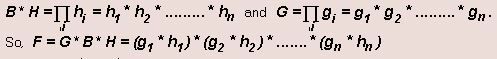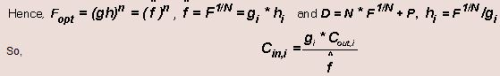In next page, we will this by an example.

Example of Minimizing delay: Consider the path from A to B involving three two input NAND gates as in fig 23.22. The input capacitance of first gate is C and the load capacitance is also C . Find the least delay in this path and how should the transistors be sized to achieve least delay?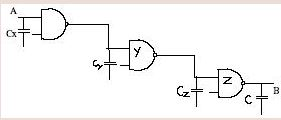Fig 23.22: Example Circuit

Solution:

Logical effort of a two input NAND gate is g = 4/3
so G = (4/3)*3 = 64/27 = 2.37 .

B = 1 (as there is no branching) , H = Cout / Cin = 1

Path Effort F = 64/27*1*1 = 64/27

if each stage has same parasitic delay then P = p1+ p2+p3 =6 pinv ( as all are two input), then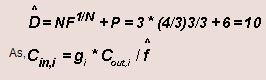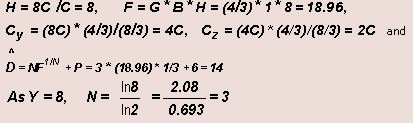23.3 Reduction of Delay

For the minimum delay of the circuit we optimizes the number of stages. Let total number of stages be N = n1 + n2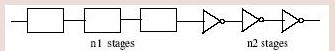Fig 23.31: Example Circuit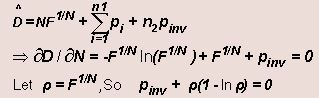But the number of stages for minimum delay may not be the integer ,so it is not feasible to implement it . So we realise the circuit by either taking the number of stages greatest integer of the obtained value or the one more then the greatest integer whatever gives us the minimun delay .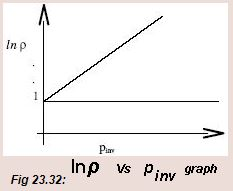Offer running on EduRev: Apply code STAYHOME200 to get INR 200 off on our premium plan EduRev Infinity!

,

,

,

,

,

,

,

,

,

,

,

,

,

,

,

,

,

,

,

,

,

;